# 小学三年级数学口算练习石家庄奥数网 数学要从娃娃抓起！小学三年级开始就要着手为以后参加全国或地方的各大杯赛做准备了，数学基础一定要打牢固，下面是三年级数学练习题，请大家做个参考。

1、三年级两位数加两位数的加法（含和超过一百的）

（1）11+18=

（2）40+49=

（3）46+38=

（4）25+57=

（5）82+58=

（6）22+91=

（7）32+43=

（8）49+73=

（9）86+33=

2、三年级三位数加三位数的加法（含连续进位的）

（1）124+895=

（2）568+370=

（3）651+662=

（4）792+799=

（5）981+728=

（6）179+525=

（7）141+912=

（8）145+913=

（9）782+335=

3、三年级三位数减三位数的减法（含连续退位的）

（1）536-461=

（2）727-157=

（3）371-116=

（4）873-416=

（5）854-247=

（6）597-184=

（7）814-279=

（8）826-523=

（9）205-164=

4、三年级整百数减三位数的减法

（1）400-164=

（2）900-546=

（3）500-120=

（4）500-171=

（5）500-115=

（6）900-614=

（7）700-133=

（8）600-333=

（9）300-196=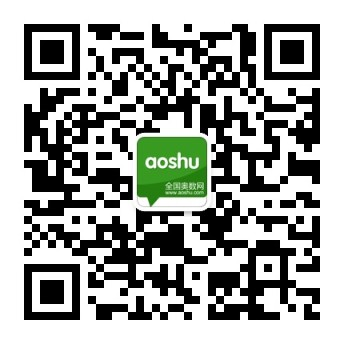您还可以通过手机、平板电脑等移动设备访问石家庄奥数网，升学路上有我们相伴。>>[点击查看]

•欢迎扫描二维码
关注奥数网微信
ID：aoshu_2003

•欢迎扫描二维码
关注中考网微信
ID：zhongkao_com

### 教育导航

1.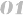北京站 上海站 广州站 深圳站
2.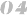天津站 武汉站 成都站 石家庄站
3.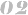南京站 杭州站 济南站 苏州站
4.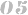郑州站 沈阳站 太原站 重庆站
5.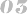长沙站 合肥站 宁波站 青岛站